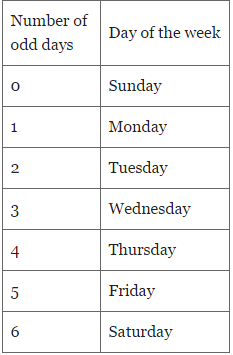LR  >  Calendars - Important Formulas; Logical Reasoning

# Calendars - Important Formulas; Logical Reasoning Notes - LR

## Document Description: Calendars - Important Formulas; Logical Reasoning for LR 2022 is part of Calendars for preparation. The notes and questions for Calendars - Important Formulas; Logical Reasoning have been prepared according to the LR exam syllabus. Information about Calendars - Important Formulas; Logical Reasoning covers topics like and Calendars - Important Formulas; Logical Reasoning Example, for LR 2022 Exam. Find important definitions, questions, notes, meanings, examples, exercises and tests below for Calendars - Important Formulas; Logical Reasoning.

Introduction of Calendars - Important Formulas; Logical Reasoning in English is available as part of our for LR & Calendars - Important Formulas; Logical Reasoning in Hindi for course. Download more important topics related with Calendars, notes, lectures and mock test series for LR Exam by signing up for free. LR: Calendars - Important Formulas; Logical Reasoning Notes - LR
 1 Crore+ students have signed up on EduRev. Have you?

Important Formulas - Calendar

1. Odd Days

Number of days more than the complete weeks are called odd days in a given period.

2. Leap Year

A leap year has 366 days.

In a leap year, the month of February has 29 days.

Every year divisible by 4 is a leap year, if it is not a century.

Examples:
1952, 2008, 1680 etc. are leap years.
1991, 2003 etc. are not leap years

Every 4th century is a leap year and no other century is a leap year.

Examples:
400, 800, 1200 etc. are leap years.
100, 200, 1900 etc. are not leap years

3. Ordinary Year

The year which is not a leap year is an ordinary year.

An ordinary year has 365 days

4. Counting odd days and calculating day of any particular date

1 ordinary year ≡ 365 days ≡ (52 weeks + 1 day)
Hence number of odd days in 1 ordinary year= 1.

1 leap year ≡ 366 days ≡ (52 weeks + 2 days)
Hence number of odd days in 1 leap year= 2.

100 years ≡ (76 ordinary years + 24 leap years )
≡ (76 x 1 + 24 x 2) odd days
≡ 124 odd days.
≡ (17 weeks + 5 days)
≡  5 odd days.

Hence number of odd days in 100 years = 5.

Number of odd days in 200 years = (5 x 2) = 10 ≡ 3 odd days

Number of odd days in 300 years = (5 x 3) = 15 ≡ 1 odd days

Number of odd days in 400 years = (5 x 4 + 1) = 21 ≡ 0 odd days

Similarly, the number of odd days in all 4th centuries (400, 800, 1200 etc.) = 0

Mapping of the number of odd day to the day of the weekLast day of a century cannot be Tuesday or Thursday or Saturday.

For the calendars of two different years to be the same, the following conditions must be satisfied.

(1) Both years must be of the same type. i.e., both years must be ordinary years or both years must be leap years.

(2) 1st January of both the years must be the same day of the week.

The document Calendars - Important Formulas; Logical Reasoning Notes - LR is a part of LR category.
All you need of LR at this link: LR
 Use Code STAYHOME200 and get INR 200 additional OFF

Track your progress, build streaks, highlight & save important lessons and more!

,

,

,

,

,

,

,

,

,

,

,

,

,

,

,

,

,

,

,

,

,

;